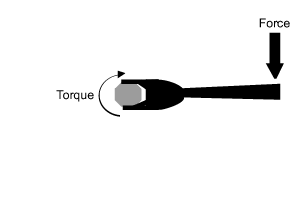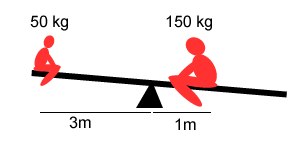Torque Any time we turn a door knob, use a wrench to tighten a bolt, or push down on a lever to make it turn we exert a turning force. A turning force produces a torque. But force is not a torque. Force causes something to accelerate or move but a torque causes something to rotate. Consider the animation on the right. Even though the force remains constant the torque gets less as the force moves closer to the turning point. A long handled wrench makes it easier to unscrew tight bolts. This is because we increase the torque by moving the applied force further from the turning point. Note, it is only the perpendicular component of the force acting on the lever that produces torque.torque = force X distance from turning point. Now we can see that using a constant force we can produce more or less torque. Using a constant applied force describe how we can increase torque. Torques exist on both sides of this lever and they are balanced.Home